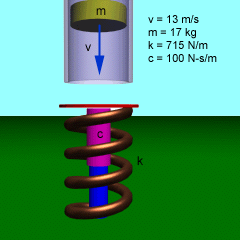Ch 10. Vibrations Multimedia Engineering Dynamics Free Vibs. Undamped Free Vibs. Damped Forced Vibration Energy Method
 Chapter - Particle - 1. General Motion 2. Force & Accel. 3. Energy 4. Momentum - Rigid Body - 5. General Motion 6. Force & Accel. 7. Energy 8. Momentum 9. 3-D Motion 10. Vibrations Appendix Basic Math Units Basic Equations Sections Search eBooks Dynamics Fluids Math Mechanics Statics Thermodynamics Author(s): Kurt Gramoll ©Kurt GramollDYNAMICS - CASE STUDY IntroductionProblem Diagram Drop-tubes are used to simulate weightlessness for various experiments. The experiment container is stopped at the bottom of the tube by a spring and damper. Since the equipment can be quite delicate, the deceleration of the container, and hence its motion, is very important. What is known: container mass m = 17 kg impact velocity v = 13 m/s spring constant k = 715 N/m damper constant c = 100 N-s/m Question What is the motion of the container after it impacts the spring and damper at the bottom of the tube? Approach Determine the differential equation of motion. Solve the equation of motion for the displacement. Apply the initial conditions.

Practice Homework and Test problems now available in the 'Eng Dynamics' mobile app
Includes over 400 problems with complete detailed solutions.
Available now at the Google Play Store and Apple App Store.